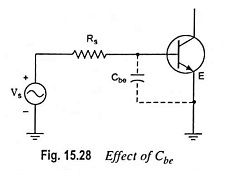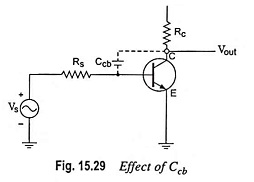## Effect of Internal Transistor Capacitance:

As we know already that at high frequencies, the coupling and bypass capacitors act as short circuit due to their low reactance and hence the voltage gain is not affected. However, the internal Transistor capacitance play vital role. They reduce the amplifier gain and introduce unwanted phase shift with the increase in frequency.

Figure 15.27 shows the internal P-N junction capacitances for a BJT. Ccb is the collector-to-base junction capacitance whereas Cbe is the base-emitter junction capacitance. These internal Transistor capacitance are in pF range and have a very high reactance at low frequencies and, therefore, they are equivalent to open circuit and do not affect the voltage gain at low frequencies. But with the increase in frequency of operation, the reactance reduces and affects the voltage gain considerably.At high frequencies, the reactance of Cbe becomes very small, a considerable portion of input signal is lost due to low voltage developed across the capacitance Cbe as shown in Fig. 15.28. The result is that the amplifier output voltage and voltage gain are reduced.At high frequencies, the reactance of Ccb becomes very small and a large portion of output voltage is fed back to the base. This feedback voltage, being out of phase with input signal Vs, reduces the effective input to the amplifier. The ultimate result is that the output voltage and voltage gains are reduced. The effect of Ccb is illustrated in Fig. 15.29.Thus the internal Transistor capacitance reduce the amplifier gain at high frequencies.

Similarly, the internal capacitances of JFET amplifiers reduce the output voltage and gain.

Scroll to Top